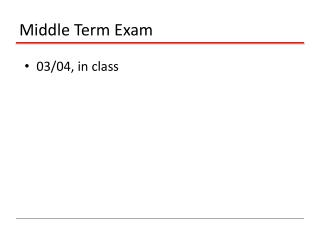# Middle Term Exam - PowerPoint PPT PresentationDownload PresentationMiddle Term Exam

Middle Term Exam
Download Presentation## Middle Term Exam

- - - - - - - - - - - - - - - - - - - - - - - - - - - E N D - - - - - - - - - - - - - - - - - - - - - - - - - - -
##### Presentation Transcript

1. Middle Term Exam 03/04, in class

2. Project • It is a team work • No more than 2 people for each team • Define a project of your own • Otherwise, I will assign you to a “tough” project • Important date • 03/23: project proposal • 04/27 and 04/29: presentation • 05/02: final report

3. Project Proposal Introduction: describe the research problem Related wok: describe the existing approaches and their deficiency Proposed approaches: describe your approaches and its potential to overcome the shortcomings of existing approaches Plan: the plan for this project (code development, data sets, and evaluation) Format: it should look like a research paper The required format (both Microsoft Word and Latex) can be downloaded from www.cse.msu.edu/~cse847/assignments/format.zip Warning: any submission that does not follow the format will be given zero score.

4. Project Report • The same format as the proposal • Expand the proposal with detailed description of your algorithm and evaluation results • Presentation • 25 minute presentation • 5 minute discussion

5. Information • Information  knowledge • Information: reduction in uncertainty • Example: • flip a coin • roll a die • #2 is more uncertain than #1 • Therefore, more information is provided by the outcome of #2 than #1

6. Definition of Information • Let E be some event that occurs with probability P(E). If we are told that E has occurred, then we say we have received I(E)=log2(1/P(E)) bits of information • Example: • Result of a fair coin flip (log22=1 bit) • Result of a fair die roll (log26=2.585 bits)

7. Entropy A zero-memory information source S is a source that emits symbols from an alphabet {s1, s2,…, sk} with probability {p1, p2,…,pk}, respectively, where the symbols emitted are statistically independent. Entropy is the average amount of information in observing the output from S

8. Entropy • 0  H(P)  logk • Measures the uniformness of a distribution P: The further P is from uniform, the lower the entropy. • For any other probability distribution {q1,…,qk},

9. A Distance Measure Between Distributions Kullback-Leibler distance between distributions P and Q 0  D(P, Q) The smaller D(P, Q), the more Q is similar to P Non-symmetric: D(P, Q)  D(Q, P)

10. Mutual Information Indicate the amount of information shared between two random variables Symmetric: I(X;Y) = I(Y;X) Zero iff X and Y are independent

11. Maximum Entropy Rong Jin

12. Motivation • Consider a translation example • English ‘in’  French {dans, en, à, au-cours-de, pendant} • Goal: p(dans), p(en), p(à), p(au-cours-de), p(pendant) • Case 1: no prior knowledge on translation • Case 2: 30% of times either dans or en is used

13. Maximum Entropy Model: Motivation • Case 3: 30% of time dans or en is used, and 50% of times dans or à is used • Need a measure the uninformness of a distribution

14. Maximum Entropy Principle (MaxEnt) • p(dans) = 0.2, p(a) = 0.3, p(en)=0.1 • p(au-cours-de) = 0.2, p(pendant) = 0.2

15. MaxEnt for Classification Objective is to learn p(y|x) Constraints Appropriate normalization

16. MaxEnt for Classification Constraints Consistent with data Feature function Model mean of feature functions Empirical mean of feature functions

17. MaxEnt for Classification No assumption about p(y|x) (non-parametric) Only need the empirical mean of feature functions

18. MaxEnt for Classification Feature function

19. Example of Feature Functions

20. Solution to MaxEnt • Identical to conditional exponential model • Solve W by maximum likelihood estimation

21. Iterative Scaling (IS) Algorithm • Assume

22. Iterative Scaling (IS) Algorithm • Compute the empirical mean for every feature and every class • Initialize • Repeat • Compute p(y|x) for each training example (xi, yi) using W • Compute the model mean of every feature for every class • Update W

23. Iterative Scaling (IS) Algorithm • It guarantees that the likelihood function always increases

24. Iterative Scaling (IS) Algorithm • How about features that can take both positive and negative values? • How about the sum of features is not a constant?

25. MaxEnt for Classification

26. MaxEnt for Classification